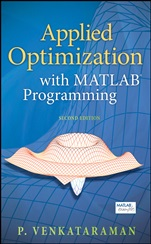### Logowanie

Zapomniałeś hasła?
Nowy klient

Jeżeli nie znalazłeś poszukiwanej książki, skontaktuj się z nami wypełniając formularz kontaktowy.

Ta strona używa plików cookies, by ułatwić korzystanie z serwisu. Mogą Państwo określić warunki przechowywania lub dostępu do plików cookies w swojej przeglądarce zgodnie z polityką prywatności.

### Informacje szczegółowe o książce# Applied Optimization with MATLAB Programming

##### ISBN 9780470084885

Autor: P. Venkataraman

Wydawca: Wiley

Dostępność: 3-6 tygodni

Cena: 757,05 zł

 ISBN13: 9780470084885 ISBN10: 047008488X Autor: P. Venkataraman Oprawa: Hardback Rok Wydania: 2009-04-03 Numer Wydania: 2nd Edition Ilość stron: 544 Wymiary: 243x166 Tematy: TG

Technology/Engineering/Mechanical
Provides all the tools needed to begin solving optimization problems using MATLAB®
The Second Edition of Applied Optimization with MATLAB® Programming enables readers to harness all the features of MATLAB® to solve optimization problems using a variety of linear and nonlinear design optimization techniques. By breaking down complex mathematical concepts into simple ideas and offering plenty of easy–to–follow examples, this text is an ideal introduction to the field. Examples come from all engineering disciplines as well as science, economics, operations research, and mathematics, helping readers understand how to apply optimization techniques to solve actual problems.
This Second Edition has been thoroughly revised, incorporating current optimization techniques as well as the improved MATLAB® tools. Two important new features of the text are:
Introduction to the scan and zoom method, providing a simple, effective technique that works for unconstrained, constrained, and global optimization problems
New chapter, Hybrid Mathematics: An Application, using examples to illustrate how optimization can develop analytical or explicit solutions to differential systems and data–fitting problems
Each chapter ends with a set of problems that give readers an opportunity to put their new skills into practice. Almost all of the numerical techniques covered in the text are supported by MATLAB® code, which readers can download on the text′s companion Web site www.wiley.com/go/venkat2e and use to begin solving problems on their own.
This text is recommended for upper–level undergraduate and graduate students in all areas of engineering as well as other disciplines that use optimization techniques to solve design problems.

Spis treści:
Preface to the Second Edition.
Preface.
Chapter 1: Introduction.
1.1 Optimization Fundamentals.
1.2 Introduction to MATLAB.
Problems.
Chapter 2: Graphical Optimization.
2.1 Problem Definition.
2.2 Graphical Solution.
References.
Problems.
Chapter 3: Linear Programming.
3.1 Problem Definition.
3.2 Graphical Solution.
3.3 Numerical Solution – The Simplex Method.
References.
Problems.
Chapter 4: Nonlinear Programming.
4.1 Problem Definition.
4.2 Mathematical Concepts.
4.3 Analytical Conditions.
4.4 Examples.
References.
Problems.
Chapter 5: Numerical Techniques – The One Dimensional Problem.
5.1 Problem Definition.
5.2 Numerical Techniques.
5.3 Importance of the One Dimensional Problem.
References.
Problems.
Chapter 6: Numerical Techniques for Unconstrained Optimization.
6.1 Problem Definition.
6.2 Numerical Techniques: Non Gradient Methods.
6.3 Numerical Technique: Gradient Based Methods.
6.4 Numerical Technique: Second Order.
6.6 Summary.
References.
Problems.
Chapter 7: Numerical Techniques for Constrained Optimization.
7.1 Problem Definition.
7.2 Indirect Methods for Constrained Optimization.
7.3 Direct Methods for Constrained Optimization.
References.
Problems.
Chapter 8: Discrete Optimization.
8.1 Concepts in Discrete Programming.
8.2 Discrete Optimization Techniques.
References.
Problems.
Chapter 9: Global Optimization.
9.1 Problem Definition.
9.2 Numerical Techniques and Additional Examples.
References.
Problems.
Chapter 10: Optimization Toolbox from MATLAB.
10.1 The Optimization Toolbox.
10.2 Examples.
References.
Ch apter 11: Hybrid Mathematics: An Application of.
11.1 Central Idea.
11.2 Data Handling Examples.
11.3. Solutions to Differential Systems.
11.4 Summary.
References.
Index.

Nota biograficzna:

P. Venkataraman, PhD, is an associate professor in the Mechanical Engineering Department, Rochester Institute of Technology, Rochester, New York.

Technology/Engineering/Mechanical
Provides all the tools needed to begin solving optimization problems using MATLAB®
The Second Edition of Applied Optimization with MATLAB® Programming enables readers to harness all the features of MATLAB® to solve optimization problems using a variety of linear and nonlinear design optimization techniques. By breaking down complex mathematical concepts into simple ideas and offering plenty of easy–to–follow examples, this text is an ideal introduction to the field. Examples come from all engineering disciplines as well as science, economics, operations research, and mathematics, helping readers understand how to apply optimization techniques to solve actual problems.
This Second Edition has been thoroughly revised, incorporating current optimization techniques as well as the improved MATLAB® tools. Two important new features of the text are:
Introduction to the scan and zoom method, providing a simple, effective technique that works for unconstrained, constrained, and global optimization problems
New chapter, Hybrid Mathematics: An Application, using examples to illustrate how optimization can develop analytical or explicit solutions to differential systems and data–fitting problems
Each chapter ends with a set of problems that give readers an opportunity to put their new skills into practice. Almost all of the numerical techniques covered in the text are supported by MATLAB® code, which readers can download on the text′s c ompanion Web site www.wiley.com/go/venkat2e and use to begin solving problems on their own.
This text is recommended for upper–level undergraduate and graduate students in all areas of engineering as well as other disciplines that use optimization techniques to solve design problems.

### Koszyk

Książek w koszyku: 0 szt.

Wartość zakupów: 0,00 zł

### Kontakt

Gambit
Centrum Oprogramowania
i Szkoleń Sp. z o.o.

Al. Pokoju 29b/22-24

31-564 Kraków

+48 12 414 3791

+48 12 414 3387

Siedziba Księgarni

ul. Kordylewskiego 1

31-542 Kraków

+48 12 410 5989

+48 12 414 3767

Wyślij e-mail

### Subskrypcje

Administratorem danych osobowych jest firma Gambit COiS Sp. z o.o. Na podany adres będzie wysyłany wyłącznie biuletyn informacyjny.

### Autoryzacja płatności